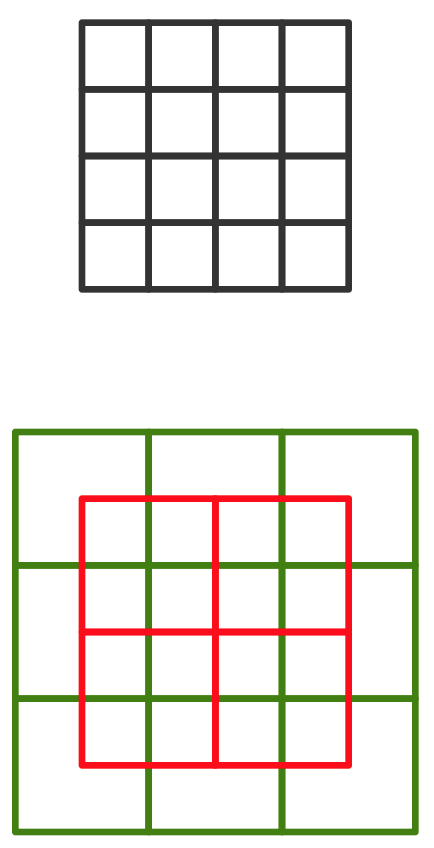## Sunday, 8 March 2015

### Coarse code representation in brain

Although neurons can be considered having active state or not, but brain at its bare neocortex layer  doesn't understand binary information or processing binary data. I mean the neocortex, for example doesn't represent a number like 6 as 0110 and ... It seems that the coarse coding is the way brain understands things, numbers, shapes, images, ... all has a coarse code representation.

Let us see how it works, first numbers. What we had and described in the previous example doesn't work here because our brain is not a binary processor, although we understand this kind of processing the understanding is a knowledge we have achieved via a hierarchical memory. So the simplest way will be like what we described in "Understanding Coarse Codes". Suppose we want to represent numbers from 0 to 9,999, we can do it by allocation 10,000 neurons each representing a number, but this is not that efficient. From the post we mentioned we have:

Number of neurons in u-v (fine resolution) coordinates: 10,000
Single axis high resolution: √100,000 = 100
Single axis low resolution : 5 // we simply consider it
Number of coordinates: Round(100/5) = 20
Number of neurons in low resolution coordinates: 20 * (5*5) = 500
Efficiency = 10,000/500 = 20 or 2,000%

So to represent 0 to 9,999 we need 500 neurons, in the form of 20 overlapped layer of coordinates each having 25 square or neurons. So just with 500 neurons having maximum active of 20 neurons which gives 20/500=4% we can identify a number between 0 to 99,999.

## Thursday, 5 March 2015

### Converting numeric data to coarse codedense vs coarse codefor 16 bits number in dense and equivalent 13 bits coarse code.
OK, suppose you have a single variable that represents the current value of a sensor. A network usage traffic, the heat bitrate of a human and ... at each time when you read the sensor value you just get a numeric number, suppose it is an integer number in the interval [0,65535] . If you want to store and process it in a dense format you need 16 bits (or neurons) to show the sensor output in dense binary format.

Let us get back to the previous post and represent the 16 bits number as a 4 × 4 square, like the picture. So with this 16 bits memory page, we can show numbers from 0 to 65,535. As you may remember if we want to use coarse coding to represent these 16 bits we need a total of 13 bits, as shown in the picture. Note that as the size of the pages get larger and memory grows the efficiency of gets better and better. But for now, forget about the efficiency, just try to understand how it works.

Where is the problem?
The problem is our storage systems or models are built/designed to store dense binary data. Even our imaginations digest and understand these kinds of storages better. So we have no choice other than figuring out a way to represent this 13 bits in an ordinary dense binary system. This may also cost us some coding and decoding procedures too.

## Tuesday, 3 March 2015

### Understanding Coarse Codes

It turns out that human brain uses coarse coding to store information it gathers. In fact, this might be the easiest way to store this huge amount of information we gather as we explore the world and live through our lives. In a nutshell, we just store specifications of a the things we see or hear or touch and ... There are neurons in our neocortex each related to any of the concepts our senses understand like big, small, square, circle, red, green, hot, cold and ... The corresponding neurons of these concepts are linked to each other and build something like a Bayesian Network, there are even some mechanisms for the conditions and probability for moving on network edges!

Coarse coding helps the brain to have accuracy when measuring or comparing things, in fact if we have 10 thousands of concept related neurons to figure out a seen or voice or ... we may just need to set 10 or 100 or 200 of these neurons active not more, we will talk about these in later posts.

## Sunday, 1 March 2015

### More about SDR and brain memory

We saw we can represent a neuron memory layer as a matrix like the following:

[
[a11, a12, ... , a1n],
[a21, a22, ... , a2n],
.
.
.
[am1, am2, ... , amn]
]

And since it is a bit difficult to show it as an m × n matrix in web we prefer to use the following one row form:

[a11, a12, ... , a1n, an+1, an+2,... , am×n]

Human brain stores information in the form of sparse storage, not dense. So you have for example a 10,000 bit of information which only small portion of them, perhaps maximum 5% are active. Each neuron represents a bit of information.